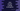# Java program to convert a string to ArrayList## Java program to convert a string to ArrayList:

In this post, we will learn how to convert a string to an ArrayList in Java. One string with comma-separated words is given. This program will take this string as input and put all words in an ArrayList. The string words could be separated by any other character other than a comma.

The idea is to split the string by words and put those words in an ArrayList.

Let’s take a look at the algorithm that we will use in this program.

### Algorithm to convert a string to ArrayList:

This program will use the below algorithm:

• Take the string in a variable and the separator  in a different variable that is separating the words in the string
• Split the words in the string and put these words in an array.
• Convert the array to an ArrayList

For the second step, we can use the split() method. This method splits the words in a string and puts them in an array.

### Java Program:

Below is the complete Java program that converts a string to an ArrayList.

``````import java.util.Arrays;
import java.util.List;

public class Main {
public static void main(String[] args) {
String givenString = "one,two,three,four,five";
String separator = ",";

List<String> arrayList;

String[] strArray = givenString.split(separator);
arrayList = Arrays.asList(strArray);

arrayList.forEach(System.out::println);
}
}``````

Here,

• givenString is the given string with words are separated by a comma.
• separator is the separator of the words i.e. comma
• arrayList variable is to store the ArrayList.
• We are using split method of String and passing the separator to it to get the words in an array. This value is stored in strArray.
• Next, we are using Arrays.asList to convert the array to an ArrayList. The last line is using *forEach *with the arraylist to iterate over the items and printing them all.

It will give result as like below:

### Removing initial and trailing blank spaces while splitting:

We can also remove the *initial *and trailing blank spaces while splitting the string.

Suppose, the givenString is:

``String givenString = " one, two ,  three,  four  ,    five ";``

The above program will print the below output for this string:

``````one
two
three
four
five``````

It didn’t remove the blanks from the start and end of the words. To do that, we need to write the program as like below:

``````import java.util.Arrays;
import java.util.List;

public class Main {
public static void main(String[] args) {
String givenString = " one, two ,  three,  four  ,    five ";
String separator = "\\s*,\\s*";

List<String> arrayList;

String[] strArray = givenString.trim().split(separator);
arrayList = Arrays.asList(strArray);

arrayList.forEach(System.out::println);
}
}``````

Here,

• The separator is changed to a regular expression or Regex that takes blanks on both sides of the comma as the separator. That removes all blanks from both sides of the string words.
• The trim() method removes the blanks from the start and end of the string givenString before split is used with it.
• Arrays.asList converts the array to an ArrayList similar to the above program.

It will print:

``````one
two
three
four
five``````

As you can see here, blank spaces are removed from both sides of the words.